Imagination is more important than knowledge... Albert Einstein

Guess is more important than calculation --- Knowhowacademy.com

For ACT
Number of Total Problems: 20.
FOR PRINT ::: (Book)

 Problem Num : 1 Type: None Section:Equation Theme:None Adjustment# : 0 Difficulty: 1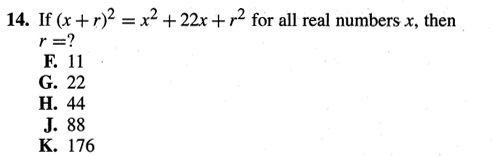Analysis

 Problem Num : 2 Type: None Section:Equation Theme:None Adjustment# : 1 Difficulty: 1Analysis

 Problem Num : 3 Type: None Section:Equation Theme:None Adjustment# : 1 Difficulty: 1Analysis factorization

 Problem Num : 4 Type: None Section:Equation Theme:None Adjustment# : 2 Difficulty: 1Analysis Use the sum of two solutions

 Problem Num : 5 Type: None Section:Equation Theme:None Adjustment# : 2 Difficulty: 1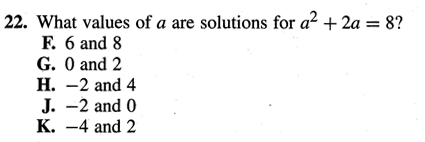Analysis

 Problem Num : 6 Type: None Section:Equation Theme:None Adjustment# : 3 Difficulty: 1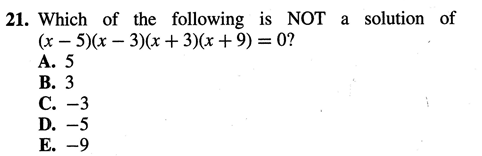Analysis

 Problem Num : 7 Type: None Section:Equation Theme:None Adjustment# : 3 Difficulty: 1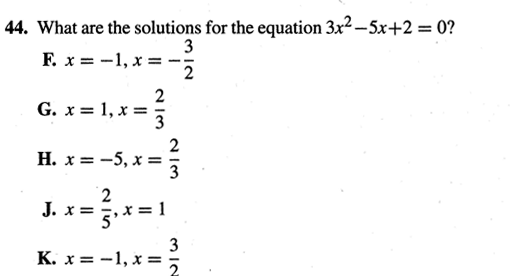Analysis Use the sum and product

 Problem Num : 8 Type: None Section:Equation Theme:None Adjustment# : 4 Difficulty: 1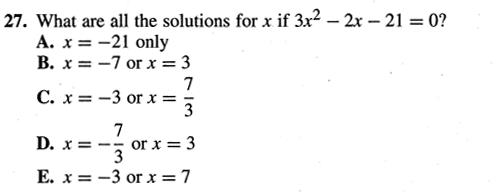Analysis

 Problem Num : 9 Type: None Section:Equation Theme:None Adjustment# : 5 Difficulty: 1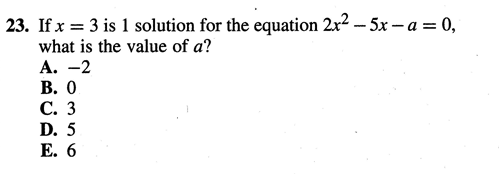Analysis There are two ways to go. Respond differently on situation.

 Problem Num : 10 Type: None Section:Equation Theme:None Adjustment# : 5 Difficulty: 1